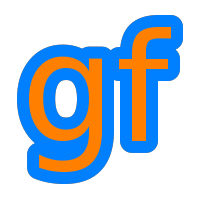Gamedev Framework (gf)  0.4.0 A C++11 framework for 2D games
gf::Polyline Class Reference

A polyline. More...

#include <gf/Polyline.h>

enum  Type {
Chain,
Loop
}

## Public Member Functions

Polyline (Type type=Chain)
Default constructor. More...

Polyline (ArrayRef< Vector2f > points, Type type=Chain)
Constructor from an array. More...

template<typename Iterator >
Polyline (Iterator first, Iterator last, Type type=Chain)
Constructor from points. More...

void addPoint (Vector2f point)
Add a point to the polyline. More...

std::size_t getPointCount () const
Get the number of points of the polyline. More...

Vector2f getPoint (std::size_t index) const
Get the i-th point of the polyline. More...

const Vector2fbegin () const
Get an iterator to the first point. More...

const Vector2fend () const
Get an iterator past the last point. More...

bool hasPrevPoint (std::size_t i) const
Check if there is a point before the i-th point. More...

Vector2f getPrevPoint (std::size_t i) const
Get the point before the i-th point. More...

bool hasNextPoint (std::size_t i) const
Check if there is a point after the i-th point. More...

Vector2f getNextPoint (std::size_t i) const
Get the point after the i-th point. More...

void setType (Type type)
Set the type of the polyline. More...

bool isLoop () const
Check is the polyline is a loop. More...

bool isChain () const
Check is the polyline is a chain. More...

## Detailed Description

A polyline.

A polyline is a set of consecutive segments. It is defined by the points ending the segments. A polyline can be open (chain) or closed (loop).

## Constructor & Destructor Documentation

 gf::Polyline::Polyline ( Type type = Chain )
inline

Default constructor.

Parameters
 type The type of polyline (default: chain)
 gf::Polyline::Polyline ( ArrayRef< Vector2f > points, Type type = Chain )
inline

Constructor from an array.

Parameters
 points The array of points type The type of polyline (default: chain)
template<typename Iterator >
 gf::Polyline::Polyline ( Iterator first, Iterator last, Type type = Chain )
inline

Constructor from points.

Parameters
 first Iterator to the first point last Iterator after the last point type The type of polyline (default: chain)

## Member Function Documentation

 void gf::Polyline::addPoint ( Vector2f point )

Add a point to the polyline.

Parameters
 point The point to add to the polyline
 const Vector2f* gf::Polyline::begin ( ) const

Get an iterator to the first point.

Returns
A pointer to the first point
end()
 const Vector2f* gf::Polyline::end ( ) const

Get an iterator past the last point.

Returns
A pointer past the last point
begin()
 Vector2f gf::Polyline::getNextPoint ( std::size_t i ) const

Get the point after the i-th point.

Parameters
 i The index of the current point
Returns
The point after the current point
hasNextPoint()
 Vector2f gf::Polyline::getPoint ( std::size_t index ) const

Get the i-th point of the polyline.

Parameters
 index The index of the point
 std::size_t gf::Polyline::getPointCount ( ) const

Get the number of points of the polyline.

Returns
The number of points of the polyline
 Vector2f gf::Polyline::getPrevPoint ( std::size_t i ) const

Get the point before the i-th point.

Parameters
 i The index of the current point
Returns
The point before the current point
hasPrevPoint()
 bool gf::Polyline::hasNextPoint ( std::size_t i ) const

Check if there is a point after the i-th point.

Parameters
 i The index of the current point
Returns
True if there is a point after the current point
getNextPoint()
 bool gf::Polyline::hasPrevPoint ( std::size_t i ) const

Check if there is a point before the i-th point.

Parameters
 i The index of the current point
Returns
True if there is a point before the current point
getPrevPoint()
 bool gf::Polyline::isChain ( ) const
inline

Check is the polyline is a chain.

Returns
True if the polyline is a chain
isLoop(), setType()
 bool gf::Polyline::isLoop ( ) const
inline

Check is the polyline is a loop.

Returns
True if the polyline is a loop